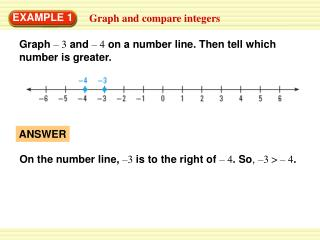# Graph and compare integers - PowerPoint PPT PresentationDownload PresentationGraph and compare integers

Presentation Description
Download Presentation## Graph and compare integers

- - - - - - - - - - - - - - - - - - - - - - - - - - - E N D - - - - - - - - - - - - - - - - - - - - - - - - - - -
##### Presentation Transcript

1. ANSWER On the number line,–3is to the right of– 4.So, –3 > – 4. EXAMPLE 1 Graph and compare integers Graph– 3and– 4on a number line. Then tell which number is greater.

2. 0 4 – 6 – 5 – 4 – 3 – 2 – 1 0 1 2 3 4 5 6 ANSWER On the number line,4is to the right of0.So, 4 > 0. for Example 1 GUIDED PRACTICE Graphthe numbers on a number line. Then tell which number is greater. 1.4and0

3. –5 2 – 6 – 5 – 4 – 3 – 2 – 1 0 1 2 3 4 5 6 ANSWER On the number line,2is to the right of–5.So, 2 > –5. for Example 1 GUIDED PRACTICE 2.2and–5

4. –1 –6 – 6 – 5 – 4 – 3 – 2 – 1 0 1 2 3 4 5 6 ANSWER On the number line,–1is to the right of–6.So, –1 > –6. for Example 1 GUIDED PRACTICE 3.–1and–6

5. Tell whether each of the following numbers is a whole number, an integer, or a rational number:5, 0.6, –2 , and –24. 2 3 Number Whole number? Integer? Rational number? 2 5 Yes Yes Yes 3 0.6 No No Yes No No Yes –2 –24 No Yes Yes EXAMPLE 2 Classify numbers

6. EXAMPLE 3 Order rational numbers ASTRONOMY A star’s color index is a measure of the temperature of the star. The greater the color index, the cooler the star. Order the stars in the table from hottest to coolest. SOLUTION Begin by graphing the numbers on a number line.

7. ANSWER From hottest to coolest, the stars are Shaula, Rigel, Denebola, and Arneb. EXAMPLE 3 Order rational numbers Read the numbers from left to right:– 0.22, – 0.03, 0.09, 0.21.

8. Number Whole number? Integer? Rational number? 3 Yes Yes Yes –1.2 No No Yes –2 No Yes Yes 0 Yes Yes Yes for Examples 2 and 3 GUIDED PRACTICE Tell whether each number in the list is a whole number, an integer, or a rational number.Then order the numbers from least to greatest. 4. 3, –1.2, –2,0

9. ANSWER –2, –1.2, 0, 3 (Ordered the numbers from least to greatest). for Examples 2 and 3 GUIDED PRACTICE

10. Number Whole number? Integer? Rational number? 5. 4.5, – , – 2.1, 0.5 4.5 No No Yes 3 No No Yes – 3 3 4 4 4 No No Yes –2 .1 0.5 No No Yes ANSWER – 2.1, – , 0.5 , – 2.1.(Order the numbers from least to greatest). for Examples 2 and 3 GUIDED PRACTICE

11. ANSWER Number Whole number? Integer? Rational number? –2.8, –1.5, – 0.31, 3.6 (Ordered the numbers from least to greatest). 3.6 No No Yes –1.5 No No Yes –0.31 No No Yes –2.8 No No Yes for Examples 2 and 3 GUIDED PRACTICE 6. 3.6, –1.5, –0.31, – 2.8

12. 2 7. , 1.75, – , 0 3 Number Whole number? Integer? Rational number? 1 No No Yes 6 1.75 No No Yes 1 1 2 6 6 No No Yes – 3 0 Yes Yes Yes ANSWER 2 – , 0 , , 1.75. (Order the numbers from leastto greatest). 3 for Examples 2 and 3 GUIDED PRACTICE

13. b.Ifa = ,then – a = – . 3 3 4 4 EXAMPLE 4 Find opposites of numbers a. Ifa=– 2.5, then –a=–(–2.5) =2.5.

14. 2 2 2 2 a.Ifa = – , then| a|= |– | = – (– )= 3 3 3 3 EXAMPLE 5 Find absolute values of numbers b.Ifa= 3.2,then|a|=|3.2|= 3.2.

15. EXAMPLE 6 Analyze a conditional statement Identify the hypothesis and the conclusion of the statement “If a number is a rational number, then the number is an integer.” Tell whether the statement is true or false. If it is false, give a counterexample. SOLUTION Hypothesis: a number is a rational number Conclusion: the number is an integer The statement is false. The number 0.5 is a counterexample, because 0.5 is a rational number but not an integer.

16. ANSWER – 5.3, 5.3 for Example 4, 5 and 6 GUIDED PRACTICE For the given value of a, find –aand |a|. 8. a = 5.3

17. ANSWER 4 4 4 9 9 9 – 10. a = 7, 7 ANSWER , for Example 4, 5 and 6 GUIDED PRACTICE 9. a = – 7

18. ANSWER Hypothesis: a number is a rational number for Example 4, 5 and 6 GUIDED PRACTICE Identify the hypothesis and the conclusion of the statement. Tell whether the statement is true or false. If it is the false, give a counterexample. 11. If a number is a rational number, then the number is positive Conclusion: the number is positive –false The number –1 is a counterexample, because –1 is a rational number but not positive.

19. 12. If the absolute value of a number is a positive, then the number is positive. ANSWER Hypothesis: the absolute value of a number is positive for Example 4, 5 and 6 GUIDED PRACTICE Conclusion: the number is positive –false The number –2 is a counterexample, because the absolute value of –2 is 2, but –2 isnegative.